##### Calculus II For DummiesSometimes the function that you’re trying to integrate is the product of two functions — for example, sin3 x and cos x. This would be simple to differentiate with the Product Rule, but integration doesn’t have a Product Rule. Fortunately, variable substitution comes to the rescue.

Given the example,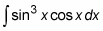1. Declare a variable as follows and substitute it into the integral:

Let u = sin x

You can substitute this variable into the expression that you want to integrate as follows: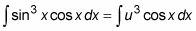Notice that the expression cos x dx still remains and needs to be expressed in terms of u.

2. Differentiate the function u = sin x.

This gives you the differential du = cos x dx.

3. Substitute du for cos x dx in the integral: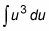4. Now you have an expression that you can integrate: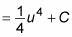5. Substitute sin x for u: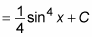Now check this answer by differentiating with the Chain Rule: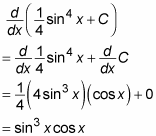This derivative matches the original function, so the integration is correct.